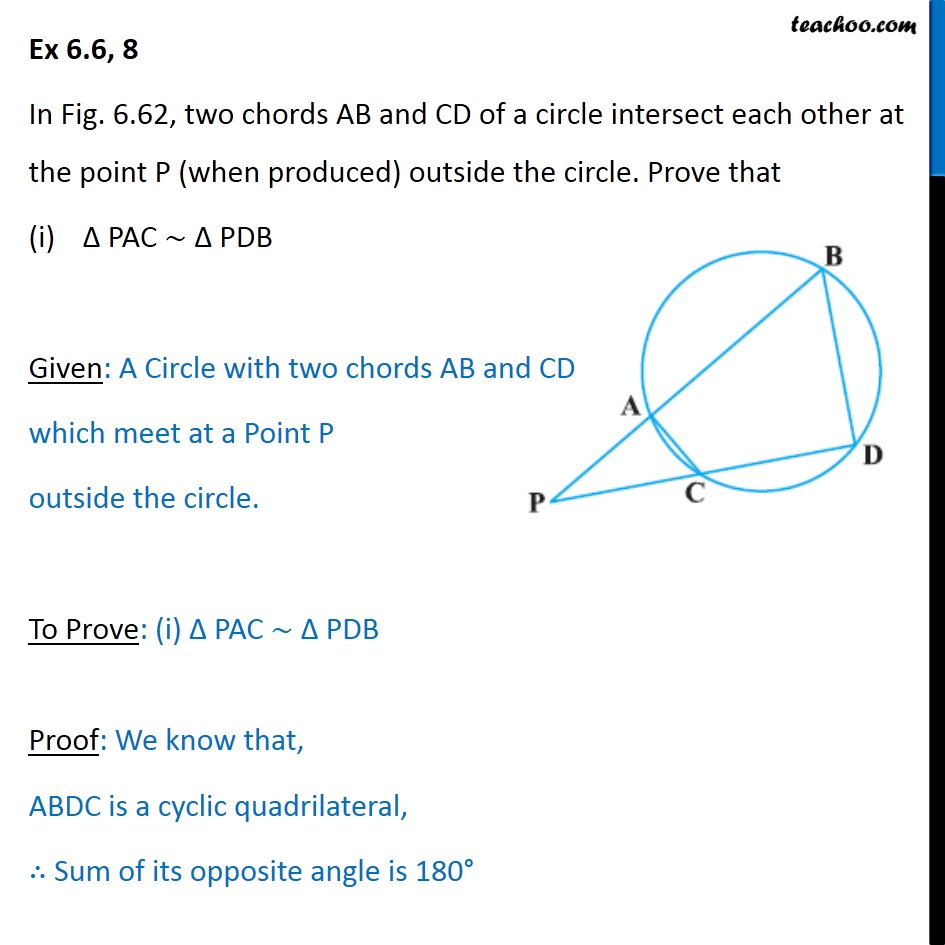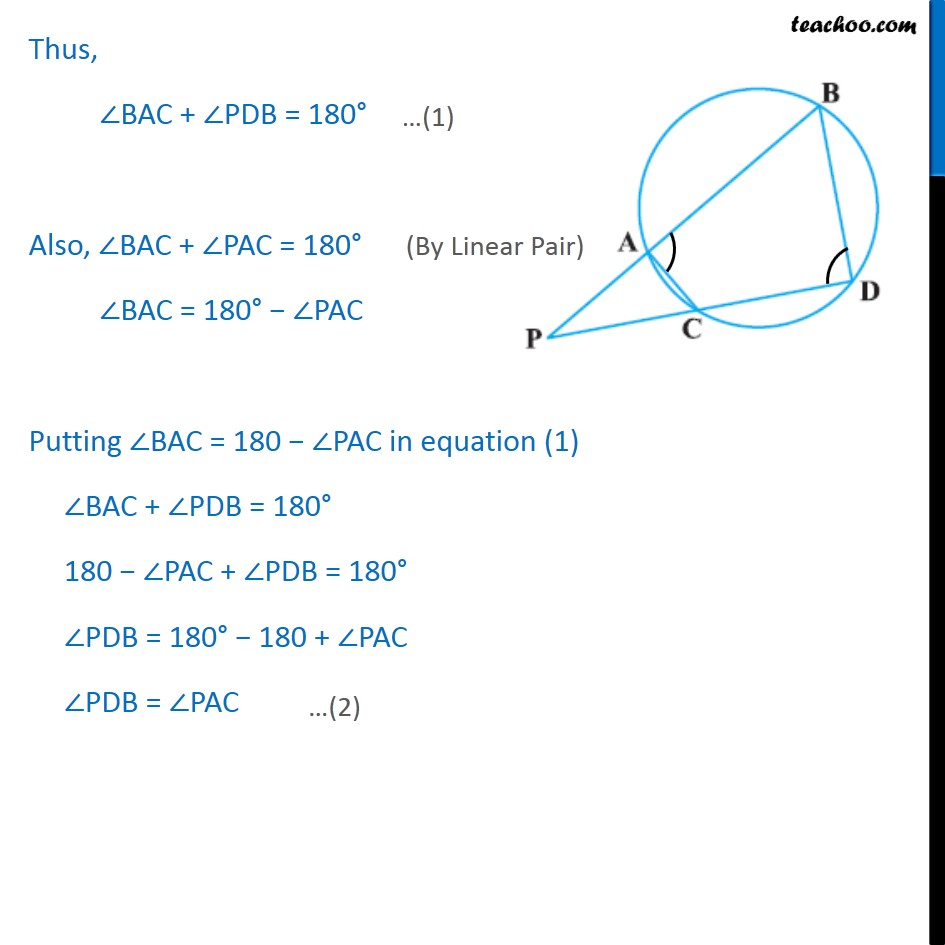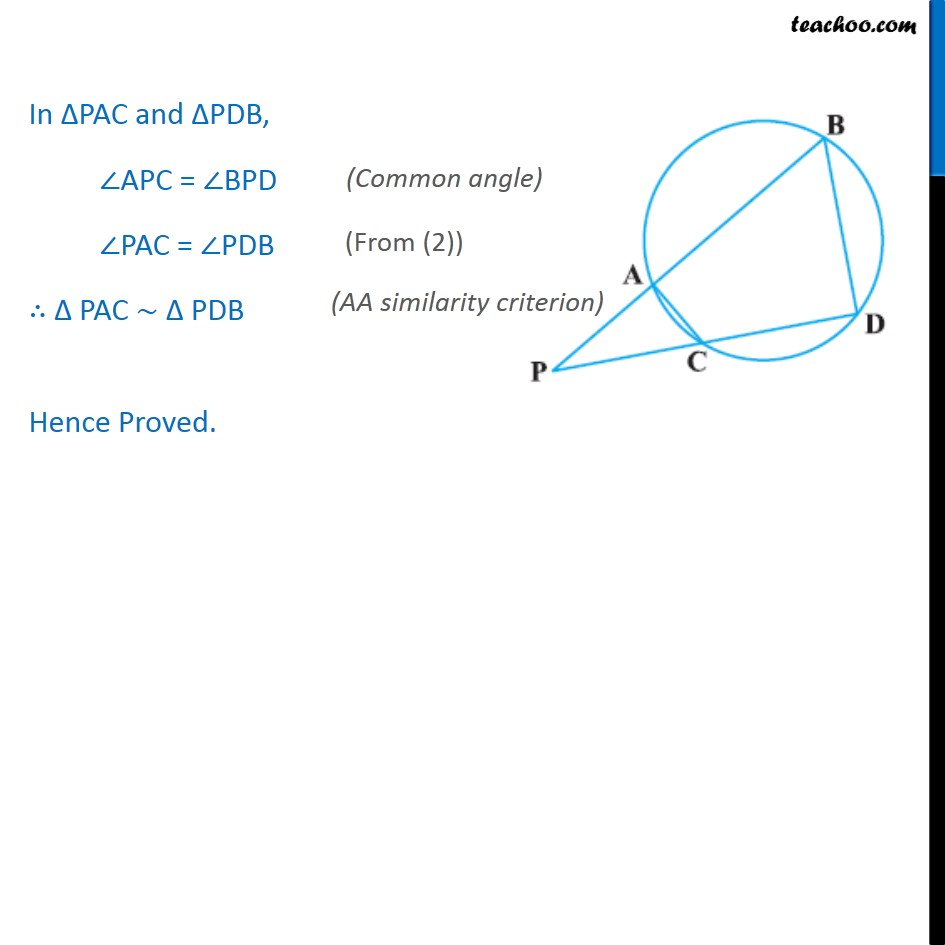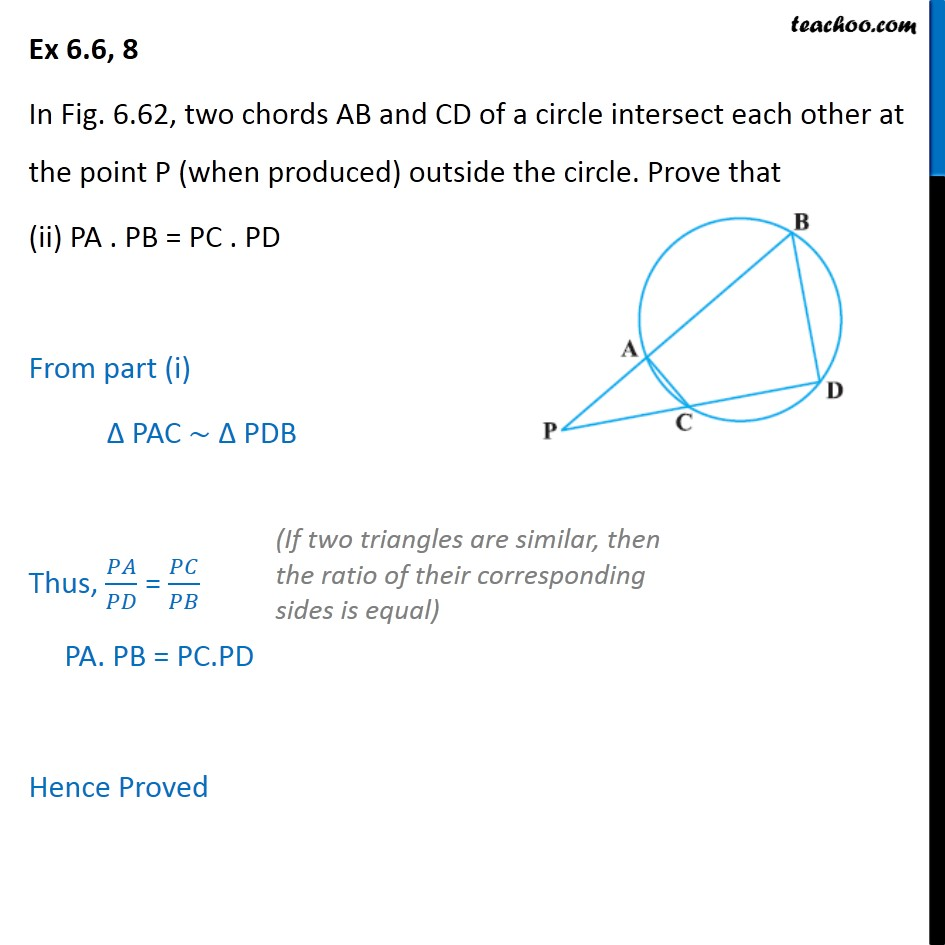Important questions of Triangle (in Geometry)

Chapter 6 Class 10 Triangles
Serial order wiseLearn in your speed, with individual attention - Teachoo Maths 1-on-1 Class

### Transcript

Question 8 In Fig. 6.62, two chords AB and CD of a circle intersect each other at the point P (when produced) outside the circle. Prove that PAC PDB Given: A Circle with two chords AB and CD which meet at a Point P outside the circle. To Prove: (i) PAC PDB Proof: We know that, ABDC is a cyclic quadrilateral, Sum of its opposite angle is 180 Thus, BAC + PDB = 180 Also, BAC + PAC = 180 BAC = 180 PAC Putting BAC = 180 PAC in equation (1) BAC + PDB = 180 180 PAC + PDB = 180 PDB = 180 180 + PAC PDB = PAC In PAC and PDB, APC = BPD PAC = PDB PAC PDB Hence Proved. Question 8 In Fig. 6.62, two chords AB and CD of a circle intersect each other at the point P (when produced) outside the circle. Prove that (ii) PA . PB = PC . PD From part (i) PAC PDB Thus, / = / PA. PB = PC.PD Hence Proved